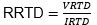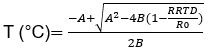# Guidelines to Implementing Temperature Measurements with the MAX22000

### 要約

This application note explains the implementation of temperature measurements provided by the MAX22000.

### Introduction

One of the important features provided by the MAX22000, besides its performance, is flexibility. Flexibility to change its operational modes and flexibility to connect different types of sensors and devices utilizing the same universal four-pin connector. Such a feature is very useful and important for industrial applications, where downtime for upgrade or reconfiguration of production lines directly affects the cost of the final products.

### Using the MAX22000 for Temperature Measurement

The MAX22000 is a highly-configurable analog I/O device, which can be transformed from a high-performing analog output device to analog input device and vice versa, or can even combine these two operational modes by proper software or firmware. The MAX22000 EV kit and its software (GUI) demonstrate these features. In this application note, we discuss implementation of temperature measurement with the resistive temperature detector (RTD) and thermocouple (TC).

### Temperature Measurement with RTD Temperature Sensor

Figure 1 shows a block diagram of the RTD connecting to the MAX22000 using a universal analog I/O connectorIn this configuration, the 4-wire RTD is excited by the current source utilizing the onboard digital-to-analog converter (DAC), current sense amplifier (CSA), and 50? current sense resistor. The voltage drop on the RTD is measured by the onboard analog-to-digital converter (ADC) through the programmable gain amplifier (PGA) and multiplexer (MUX). Such configuration allows for the length of RTD wires to be ignored due to its Kelvin connection scheme.

Flexibility of the MAX22000 configuration also allows for connection with 2-wire or 3-wire RTD. For 2-wire RTD, pins 1, 2 and 3, 4 must be shorted externally, for 3-wire RTD either pins 1 and 2 or 3 and 4 must be shorted externally.

The RTD resistance (RRTD) can be computed at any time by dividing the voltage drop on RTD (VRTD) by the exciting current (IRTD) set by the DAC (Equation 1).

Equation 1. RTD resistance calculation.The RTD temperature can be computed by using the Callendar-Van Dusen equation (Equation 2).

Equation 2. The Callendar-Van Dusen equation.
R(T) = R0(1 + A × T + B × T2 – 100 × C × T3 + C × T4)

Where,

R(T) = RTD resistance at temperature T (°C);
R0 = RTD resistance at 0°C.
A, B and C constants are derived from experimentally determined parameters and regulated by the IEC751 standard.
They also must be provided by RTD manufacturers.
For Pt100 RTD and temperature coefficient of resistance, a = 0.003850 where,
a = (R100 – R0)/(100 × R0);
A = 3.90830 × 10 - 3;
B = -5.77500 × 10 - 7;
C= 4.18301 × 10 - 12 for -200°C = T = 0°C;
C= 0 for 0°C = T = 850°C.

Temperature (T) can be calculated by solving the quadratic equation if we ignore coefficient C = 4.18301 × 10 - 12 for negative temperature (Equation 3).

Equation 3.This algorithm is implemented in the MAX22000 EV kit software and verified with the Fluke® 724 Temperature Calibrator for Pt100 for a temperature range from -200°C to 800°C, and for Pt1000 for a temperature range from -100°C to 100°C. The results are shown in the Table 1 and Table 2. The IRTD is set to 0.500006mA and PGA mode is 250mV and 2.5V respectively.

 Temperature (°C) ADC Output (mV) RRTD (?) Calculated Temperature (°C) ABS Error (°C) 800 187.81587 375.63 799.74 -0.26 600 156.82232 313.64 599.79 -0.21 400 123.52508 247.05 399.87 -0.13 200 87.91572 175.83 199.93 -0.07 100 69.24668 138.49 99.96 -0.04 50 59.69459 119.39 49.98 -0.02 0 49.99954 100.00 -0.01 -0.01 -50 40.15461 80.31 -50.01 -0.01 -100 30.13253 60.26 -100.19 -0.19 -200 9.27112 18.54 -202.37 -2.37
 Temperature (°C) ADC Output (mV) RRTD (?) Calculated Temperature (°C) ABS Error (°C) 100 692.22 1384.46 100.04 0.04 80 654.17 1308.36 80.03 0.03 60 615.92 1231.86 60.03 0.03 40 577.41 1154.84 40.02 0.02 20 538.67 1077.36 20.00 0.00 0 499.72 999.45 0.00 0.00 -20 460.51 921.03 -20.02 -0.02 -40 421.07 842.15 -40.03 -0.03 -60 381.38 762.77 -60.06 -0.06 -80 341.37 682.75 -80.13 -0.13 -100 301.03 602.07 -100.25 -0.25

The MAX absolute error of RTD measurement is below 0.3°C in a temperature range from 100°C to +800°C as shown in Table 1 and Table 2.

### Temperature Measurement with Thermocouple

Precision temperature measurement by thermocouple can be expressed by Equation 4, Equation 5, and Equation 6.

Equation 4. Voltage measured.
VM = VTC + VCOLD

Where,

VM = Measured voltage of the hot junction ends of the thermocouple;
VTC = Voltage generated by junction materials of the thermocouple relative to cold junction;
VCOLD = Cold junction voltage or reference point.
The voltage generated by the junction materials can be computed as:

Equation 5. Voltage at junction of thermocouple.
VM = VTC + VCOLD

Thus, it is required to have two independent channels of measurement – one for the hot junction and another for cold junction temperature. Figure 2 shows a block diagram for temperature measurement by the thermocouple.The hot junction temperature is measured through PGA between AI5 (pin 2) and AI6 (pin 3, 4) inputs. Cold junction temperature is measured through AUX1 and AUX2 ADC inputs by the onboard 1k RTD, Pt1000 (RT1). The Pt1000 RTD is excited by the current source set by the DAC. All calculations of the cold junction temperature are the same as explained above for the RTD measurements.

Since the common mode for AUX inputs is 1.25V, we compensate it with the 2.49k series resistor R34 and excitation current of 0.5mA.

The hot junction temperature is computed based on the thermocouple type. The most popular thermocouples for industrial applications are E, J, K, M, N, T, etc. They have different sensitivity, temperature ranges, made materials, costs and their characteristics are standardized by International Electrotechnical Commission (IEC®) and national standards: NIST (U.S.), DIN (Germany), etc.

In our example we are using emulation of a general-purpose Type-K thermocouple provided by the Fluke 724 Temperature Calibrator. It has a wide temperature range from -200°C to +1370°C with a relatively high sensitivity of about 41µV/°C.

Hot junction temperature can be computed using a look-up table from the NIST ITS-90 Thermocouple Database or Equation 5, and standardized polynomial coefficients from Table 3 for Type-K thermocouples.

Equation 6. Junction temperature in °C.
TJ = d0 + d1E + d2E2 + ... dNEN

Where,
TJ = Junction temperature in °C;
E = Computed thermocouple output by equation 5 in mV;
dN = Polynomial coefficients unique for each thermocouple type;
N = Maximum order of the polynomial.

VCOLD can be found in this table based on cold junction temperature measured by the onboard RTD (RT1) and then added to the measured VM to calculate VTC. The TJ is computed by Equation 6, where E = VTC.

 Temperature Range (°C) -200 to 0 0 to 500 500 to 1372 Voltage Range (mV) -5.891 to 1 0 to 20.644 20.644 to 54.886 Type-K TC Coefficients d0 0.0000000E+00 0.000000E+00 -1.318058E+02 d1 2.5173462E+01 2.508355E+01 4.830222E+01 d2 -1.1662878E+00 7.860106E-02 -1.646031E+00 d3 -1.0833638E+00 -2.503131E-01 5.464731E-02 d4 -8.9773540E-01 8.315270E-02 -9.650715E-04 d5 -3.7342377E-01 -1.228034E-02 8.802193E-06 d6 -8.6632643E-02 9.804036E-04 -3.110810E-08 d7 -1.0450598E-02 -4.413030E-05 0.000000E+00 d8 -5.1920577E-04 1.057734E-06 0.000000E+00 d9 0.0000000E+00 -1.052755E-08 0.000000E+00 Error Range (°C) -0.02 to 0.04 -0.05 to 0.04 -0.05 to 0.06

The result of Type-K thermocouple measurements is shown in Table 4. The IRTD is set to 0.500006mA and PGA mode is 250mV. To improve the accuracy an additional linearization of the result can be applied.

 Temperature (°C) GUI Read (mV) Error (%FS) Temperature After Linearization (°C) ABS Error After Linearization (°C) 1370 1366.66 -0.213 1370.570 0.57 1000 998.39 -0.103 1000.349 0.35 800 799.25 -0.048 800.153 0.15 600 600.04 0.003 599.887 -0.11 400 400.83 0.053 399.621 -0.38 200 201.68 0.107 199.416 -0.58 100 101.98 0.126 99.188 -0.81 50 52.29 0.146 49.234 -0.77 0 2.51 0.160 -0.810 -0.81 -50 -46.97 0.193 -50.552 -0.55 -100 -96.28 0.237 -100.123 -0.12 -200 -192.28 0.492 -196.632 3.37

### Conclusion

The Maxim Integrated MAX22000 configurable analog I/O device provides a high level of flexibility, robustness and accuracy for industrial applications, such as programmable logic controllers (PLC), programmable automation controllers (PAC), and distributed control systems (DCS). Its configurable software allows quick and seamless work with different types of sensors, in voltage or current mode, input or output mode, by enabling or disabling any of the eight available input ports.